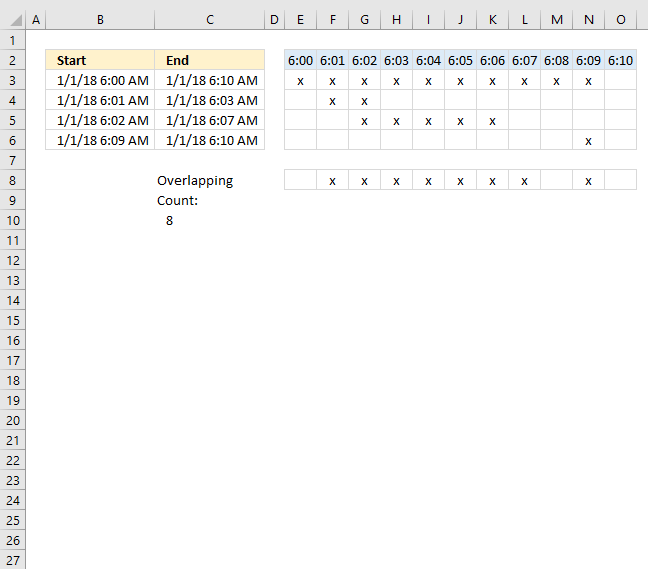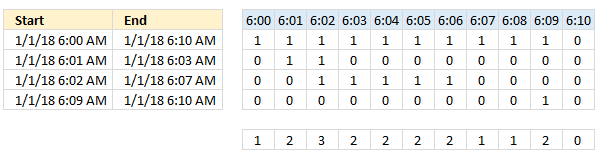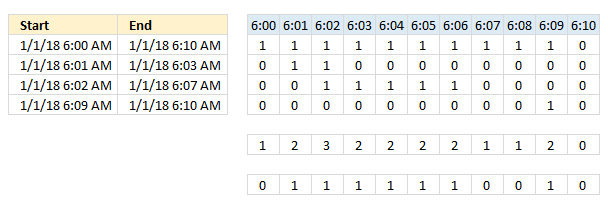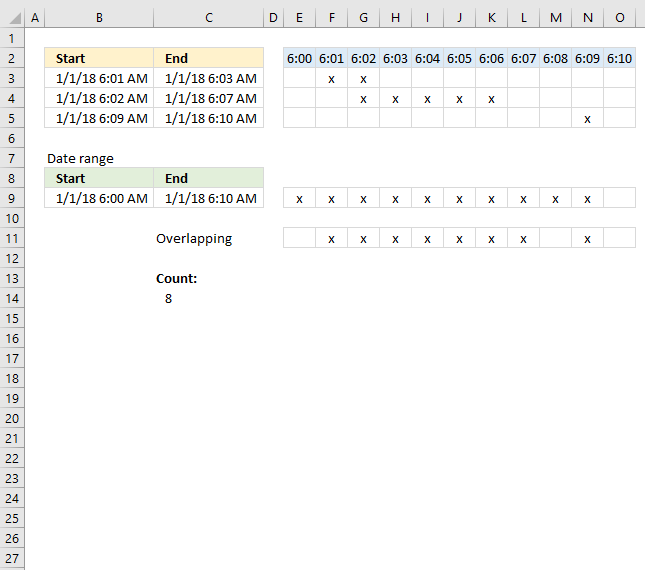Author: Oscar Cronquist Article last updated on February 01, 2023The worksheet above shows four different time ranges in column B and C, the formula in cell C10 counts the number of overlapping minutes.

The formula uses the earliest and latest date and time value in column B and C as the range to count overlapping minutes.

## 1. Sum overlapping timeArray formula in cell C10:

=SUM((MMULT(TRANSPOSE(\$B\$3:\$B\$6^0), (TRANSPOSE(MIN(\$B\$3:\$B\$6)+(ROW(A1:INDEX(\$A:\$A, (MAX(\$C\$3:\$C\$6)-MIN(\$B\$3:\$B\$6))*1440+1))-1)/1440)>=\$B\$3:\$B\$6)*((TRANSPOSE(MIN(\$B\$3:\$B\$6)+(ROW(A1:INDEX(\$A:\$A, (MAX(\$C\$3:\$C\$6)-MIN(\$B\$3:\$B\$6))*1440+1))-1)/1440))<\$C\$3:\$C\$6))>1)*1)

To enter an array formula, type the formula in a cell then press and hold CTRL + SHIFT simultaneously, now press Enter once. Release all keys.

The formula bar now shows the formula with a beginning and ending curly bracket telling you that you entered the formula successfully. Don't enter the curly brackets yourself.

### Explaining formula in cell C10

The INDEX function allows you to create an array of values, in this case, minute intervals.

MIN(\$B\$3:\$B\$6)+(ROW(A1:INDEX(\$A:\$A, (MAX(\$C\$3:\$C\$6)-MIN(\$B\$3:\$B\$6))*1440+1))-1)/1440

returns

{43101.25, 43101.2506944444, 43101.2513888889, 43101.2520833333, 43101.2527777778, 43101.2534722222, 43101.2541666667, 43101.2548611111, 43101.2555555556, 43101.25625, 43101.2569444444}

Now check if these minute intervals are between or equal to each date and time range.

(TRANSPOSE(MIN(\$B\$3:\$B\$6)+(ROW(A1:INDEX(\$A:\$A, (MAX(\$C\$3:\$C\$6)-MIN(\$B\$3:\$B\$6))*1440+1))-1)/1440)>=\$B\$3:\$B\$6)*((TRANSPOSE(MIN(\$B\$3:\$B\$6)+(ROW(A1:INDEX(\$A:\$A, (MAX(\$C\$3:\$C\$6)-MIN(\$B\$3:\$B\$6))*1440+1))-1)/1440))<\$C\$3:\$C\$6)

returns

{1, 1, 1, 1, 1, 1, 1, 1, 1, 1, 0; 0, 1, 1, 1, 0, 0, 0, 0, 0, 0, 0; 0, 0, 1, 1, 1, 1, 1, 1, 0, 0, 0; 0, 0, 0, 0, 0, 0, 0, 0, 0, 1, 0}

The MMULT function allows you to add these values column by column.

MMULT(TRANSPOSE(\$B\$3:\$B\$6^0), (TRANSPOSE(MIN(\$B\$3:\$B\$6)+(ROW(A1:INDEX(\$A:\$A, (MAX(\$C\$3:\$C\$6)-MIN(\$B\$3:\$B\$6))*1440+1))-1)/1440)>=\$B\$3:\$B\$6)*((TRANSPOSE(MIN(\$B\$3:\$B\$6)+(ROW(A1:INDEX(\$A:\$A, (MAX(\$C\$3:\$C\$6)-MIN(\$B\$3:\$B\$6))*1440+1))-1)/1440))<\$C\$3:\$C\$6))

becomes

MMULT(TRANSPOSE(\$B\$3:\$B\$6^0), {1, 1, 1, 1, 1, 1, 1, 1, 1, 1, 0; 0, 1, 1, 1, 0, 0, 0, 0, 0, 0, 0; 0, 0, 1, 1, 1, 1, 1, 1, 0, 0, 0; 0, 0, 0, 0, 0, 0, 0, 0, 0, 1, 0})

becomes

MMULT({1,1,1,1}, {1, 1, 1, 1, 1, 1, 1, 1, 1, 1, 0; 0, 1, 1, 1, 0, 0, 0, 0, 0, 0, 0; 0, 0, 1, 1, 1, 1, 1, 1, 0, 0, 0; 0, 0, 0, 0, 0, 0, 0, 0, 0, 1, 0})

and returns

{1, 2, 3, 3, 2, 2, 2, 2, 1, 2, 0}

The following picture shows the array and what the MMULT function returns.A value larger than 1 indicates an overlapping time value.

MMULT(TRANSPOSE(\$B\$3:\$B\$6^0), (TRANSPOSE(MIN(\$B\$3:\$B\$6)+(ROW(A1:INDEX(\$A:\$A, (MAX(\$C\$3:\$C\$6)-MIN(\$B\$3:\$B\$6))*1440+1))-1)/1440)>=\$B\$3:\$B\$6)*((TRANSPOSE(MIN(\$B\$3:\$B\$6)+(ROW(A1:INDEX(\$A:\$A, (MAX(\$C\$3:\$C\$6)-MIN(\$B\$3:\$B\$6))*1440+1))-1)/1440))<=\$C\$3:\$C\$6))>1

becomes

{1, 2, 3, 3, 2, 2, 2, 2, 1, 2, 0}>1

and returns

{FALSE, TRUE, TRUE, TRUE, TRUE, TRUE, TRUE, TRUE, FALSE, TRUE, FALSE}.

Lastly, multiply with 1 to convert boolean values to numerical values and then sum the numbers.

SUM((MMULT(TRANSPOSE(\$B\$3:\$B\$6^0), (TRANSPOSE(MIN(\$B\$3:\$B\$6)+(ROW(A1:INDEX(\$A:\$A, (MAX(\$C\$3:\$C\$6)-MIN(\$B\$3:\$B\$6))*1440+1))-1)/1440)>=\$B\$3:\$B\$6)*((TRANSPOSE(MIN(\$B\$3:\$B\$6)+(ROW(A1:INDEX(\$A:\$A, (MAX(\$C\$3:\$C\$6)-MIN(\$B\$3:\$B\$6))*1440+1))-1)/1440))<=\$C\$3:\$C\$6))>1)*1)

becomes

SUM({FALSE, TRUE, TRUE, TRUE, TRUE, TRUE, TRUE, TRUE, FALSE, TRUE, FALSE}*1)

becomes

SUM({0,1,1,1,1,1,1,1,0,1,0})and returns 8.

### 1.1 Sum overlapping time based on a date rangeThe picture above shows you a different setup, it allows you to use a smaller range than the min and max date and time in B3:C5.

Formula in cell C14:

=SUM((MMULT(TRANSPOSE(\$B\$3:\$B\$5^0), (TRANSPOSE(\$B\$9+(ROW(A1:INDEX(\$A:\$A, (\$C\$9-\$B\$9)*1440+1))-1)/1440)>=\$B\$3:\$B\$5)*((TRANSPOSE(\$B\$9+(ROW(A1:INDEX(\$A:\$A, (\$C\$9-\$B\$9)*1440+1))-1)/1440))<\$C\$3:\$C\$5))>0)*1)

The ROW function limits the use of these formulas, if you have a range larger than 1,048,576 minutes, which is the same as the number of rows in a worksheet, you will need another solution than the one presented here.

### Get Excel *.xlsx file

How to count overlapping timev3.xlsx

## 2. Sum overlapping time - Excel 365This Excel 365 formula works just like the formula in section 1.1 above, however, the SEQUENCE and LET functions simplify and shorten the formula considerably.

Dynamic array formula in cell C10:

=LET(y, B3:B5, x, B9+SEQUENCE(, (C9-B9)*1440+1, 0)/1440, SUM((MMULT(TRANSPOSE(y^0), (((x)>=y)*((x)<C3:C5)>0)*1)>0)*1))

### Explaining formula

The date and time values are actually numbers, dates are whole numbers. 1 is 1/1/1900 and the next day 1/2/1900 is 2 and so on. Decimal numbers are time values in Excel, for example:

00:00 AM is 0 (zero)
12:00 PM is 0.5

The whole number and the decimal number together form a date and time value in Excel. 1.5 is 1/1/1900 12:00 PM.

#### Step 1 - Subtract numbers

The minus sign lets you subtract numbers in Excel formulas, this example calculates a number representing the difference between the two date and time values specified in cells C9 and B9.

C9-B9

becomes

43101.2569444445 - 43101.25

and returns 0.00694444450346055

#### Step 2 - Multiply with 1440

The asterisk lets you multiply numbers in an Excel formula. There are 60 minutes in one hour, and twenty-four hours in one day. 60*24 equals 1440.

(C9-B9)*1440

becomes

0.00694444450346055*1440

and returns

10.0000000849832

#### Step 3 - Add 1

The plus sign lets you add numbers in an Excel formula.

(C9-B9)*1440+1

becomes

10.0000000849832+1

and returns

11.0000000849832

#### Step 4 - Create a sequence of numbers

The SEQUENCE function creates a list of sequential numbers.

Function syntax: SEQUENCE(rows, [columns], [start], [step])

SEQUENCE(,(C9-B9)*1440+1,0)

becomes

SEQUENCE(,11.0000000849832,0)

and returns

{0, 1, 2, 3, 4, 5, 6, 7, 8, 9, 10}.

SEQUENCE(,(C9-B9)*1440+1,0)/1440

becomes

{0, 1, 2, 3, 4, 5, 6, 7, 8, 9, 10}/1440

and returns

{0, 0.000694444444444444, 0.00138888888888889, 0.00208333333333333, 0.00277777777777778, 0.00347222222222222, 0.00416666666666667, 0.00486111111111111, 0.00555555555555556, 0.00625, 0.00694444444444444}

#### Step 5 - Add sequence to start date

B9+SEQUENCE(,(C9-B9)*1440+1,0)/1440

becomes

43101.25 + {0, 0.000694444444444444, 0.00138888888888889, 0.00208333333333333, 0.00277777777777778, 0.00347222222222222, 0.00416666666666667, 0.00486111111111111, 0.00555555555555556, 0.00625, 0.00694444444444444}

and returns

{43101.25, 43101.2506944444, 43101.2513888889, 43101.2520833333, 43101.2527777778, 43101.2534722222, 43101.2541666667, 43101.2548611111, 43101.2555555556, 43101.25625, 43101.2569444444}

#### Step 6 - Check if values in array is larger or equal to each value in cell range B3:B5

The larger than sign and the equal signs are logical operators, they return TRUE or FALSE if a condition is met or not.

(B9+SEQUENCE(,(C9-B9)*1440+1,0)/1440)>=B3:B5

becomes

{43101.25, 43101.2506944444, 43101.2513888889, 43101.2520833333, 43101.2527777778, 43101.2534722222, 43101.2541666667, 43101.2548611111, 43101.2555555556, 43101.25625, 43101.2569444444}>={43101.2506944444; 43101.2513888889; 43101.25625}

and returns

{FALSE, TRUE, TRUE, TRUE, TRUE, TRUE, TRUE, TRUE, TRUE, TRUE, TRUE;FALSE, FALSE, TRUE, TRUE, TRUE, TRUE, TRUE, TRUE, TRUE, TRUE, TRUE;FALSE, FALSE, FALSE, FALSE, FALSE, FALSE, FALSE, FALSE, FALSE, TRUE, TRUE}

#### Step 7 - Check if values in array is smaller to each value in cell range B3:B5

(B9+SEQUENCE(,(C9-B9)*1440+1,0)/1440)<C3:C5

becomes

{43101.25, 43101.2506944444, 43101.2513888889, 43101.2520833333, 43101.2527777778, 43101.2534722222, 43101.2541666667, 43101.2548611111, 43101.2555555556, 43101.25625, 43101.2569444444}<{43101.2520833333; 43101.2548611111; 43101.2569444445}

and returns

{TRUE, TRUE, TRUE, FALSE, FALSE, FALSE, FALSE, FALSE, FALSE, FALSE, FALSE;TRUE, TRUE, TRUE, TRUE, TRUE, TRUE, TRUE, FALSE, FALSE, FALSE, FALSE;TRUE, TRUE, TRUE, TRUE, TRUE, TRUE, TRUE, TRUE, TRUE, TRUE, TRUE}

#### Step 8 - Multiply arrays (AND logic)

The asterisk character lets you multiply numbers in an Excel formula, the equivalent function would be the PRODUCT function.

((B9+SEQUENCE(,(C9-B9)*1440+1,0)/1440)>=B3:B5)*((B9+SEQUENCE(,(C9-B9)*1440+1,0)/1440)<C3:C5)

becomes

{FALSE, TRUE, TRUE, TRUE, TRUE, TRUE, TRUE, TRUE, TRUE, TRUE, TRUE;FALSE, FALSE, TRUE, TRUE, TRUE, TRUE, TRUE, TRUE, TRUE, TRUE, TRUE;FALSE, FALSE, FALSE, FALSE, FALSE, FALSE, FALSE, FALSE, FALSE, TRUE, TRUE}*{TRUE, TRUE, TRUE, FALSE, FALSE, FALSE, FALSE, FALSE, FALSE, FALSE, FALSE;TRUE, TRUE, TRUE, TRUE, TRUE, TRUE, TRUE, FALSE, FALSE, FALSE, FALSE;TRUE, TRUE, TRUE, TRUE, TRUE, TRUE, TRUE, TRUE, TRUE, TRUE, TRUE}

and returns

{0, 1, 1, 0, 0, 0, 0, 0, 0, 0, 0;0, 0, 1, 1, 1, 1, 1, 0, 0, 0, 0;0, 0, 0, 0, 0, 0, 0, 0, 0, 1, 1}.

When you multiply boolean values TRUE or FALSE the result is their numerical equivalent:
TRUE = 1
FALSE = 0 (zero)

#### Step 9 - Check if result is larger than 0 (zero)

((B9+SEQUENCE(,(C9-B9)*1440+1,0)/1440)>=B3:B5)*((B9+SEQUENCE(,(C9-B9)*1440+1,0)/1440)<C3:C5)>0

becomes

{0, 1, 1, 0, 0, 0, 0, 0, 0, 0, 0;0, 0, 1, 1, 1, 1, 1, 0, 0, 0, 0;0, 0, 0, 0, 0, 0, 0, 0, 0, 1, 1}>0

and returns

{FALSE, TRUE, TRUE, FALSE, FALSE, FALSE, FALSE, FALSE, FALSE, FALSE, FALSE;FALSE, FALSE, TRUE, TRUE, TRUE, TRUE, TRUE, FALSE, FALSE, FALSE, FALSE;FALSE, FALSE, FALSE, FALSE, FALSE, FALSE, FALSE, FALSE, FALSE, TRUE, TRUE}.

#### Step 10 - Rearrange vertical values to horizontal values

The TRANSPOSE function converts a vertical range to a horizontal range, or vice versa.

Function syntax: TRANSPOSE(array)

TRANSPOSE(B3:B5^0)

becomes

TRANSPOSE({43101.2506944444; 43101.2513888889; 43101.25625}^0)

becomes

TRANSPOSE({1; 1; 1})

and returns

{1, 1, 1}.

#### Step 11 - Convert boolean values to their numerical equivalents

(((B9+SEQUENCE(,(C9-B9)*1440+1,0)/1440)>=B3:B5)*((B9+SEQUENCE(,(C9-B9)*1440+1,0)/1440)<C3:C5)>0)*1

becomes

{FALSE, TRUE, TRUE, FALSE, FALSE, FALSE, FALSE, FALSE, FALSE, FALSE, FALSE;FALSE, FALSE, TRUE, TRUE, TRUE, TRUE, TRUE, FALSE, FALSE, FALSE, FALSE;FALSE, FALSE, FALSE, FALSE, FALSE, FALSE, FALSE, FALSE, FALSE, TRUE, TRUE}*1

and returns

{0, 1, 1, 0, 0, 0, 0, 0, 0, 0, 0;0, 0, 1, 1, 1, 1, 1, 0, 0, 0, 0;0, 0, 0, 0, 0, 0, 0, 0, 0, 1, 1}

#### Step 12 - Calculate the matrix product of two arrays

The MMULT function calculates the matrix product of two arrays, an array as the same number of rows as array1 and columns as array2.

Function syntax: MMULT(array1, array2)

MMULT(TRANSPOSE(B3:B5^0),(((B9+SEQUENCE(,(C9-B9)*1440+1,0)/1440)>=B3:B5)*((B9+SEQUENCE(,(C9-B9)*1440+1,0)/1440)<C3:C5)>0)*1)

becomes

MMULT({1, 1, 1},{0, 1, 1, 0, 0, 0, 0, 0, 0, 0, 0;0, 0, 1, 1, 1, 1, 1, 0, 0, 0, 0;0, 0, 0, 0, 0, 0, 0, 0, 0, 1, 1})

and returns

{0, 1, 2, 1, 1, 1, 1, 0, 0, 1, 1}

#### Step 13 - Check if result is larger than 0 (zero)

MMULT(TRANSPOSE(B3:B5^0),(((B9+SEQUENCE(,(C9-B9)*1440+1,0)/1440)>=B3:B5)*((B9+SEQUENCE(,(C9-B9)*1440+1,0)/1440)<C3:C5)>0)*1)>0

becomes

{0, 1, 2, 1, 1, 1, 1, 0, 0, 1, 1}>0

and returns

{FALSE, TRUE, TRUE, TRUE, TRUE, TRUE, TRUE, FALSE, FALSE, TRUE, TRUE}.

#### Step 14 - Convert boolean values to numerical equivalents

(MMULT(TRANSPOSE(B3:B5^0),(((B9+SEQUENCE(,(C9-B9)*1440+1,0)/1440)>=B3:B5)*((B9+SEQUENCE(,(C9-B9)*1440+1,0)/1440)<C3:C5)>0)*1)>0)*1

becomes

{FALSE, TRUE, TRUE, TRUE, TRUE, TRUE, TRUE, FALSE, FALSE, TRUE, TRUE}*1

and returns

{0, 1, 1, 1, 1, 1, 1, 0, 0, 1, 1}.

#### Step 15 - Add numbers in array and return a total

The SUM function allows you to add numerical values, the function returns the sum in the cell it is entered in. The SUM function is cleverly designed to ignore text and boolean values, adding only numbers.

Function syntax: SUM(number1, [number2], ...)

SUM((MMULT(TRANSPOSE(B3:B5^0),(((B9+SEQUENCE(,(C9-B9)*1440+1,0)/1440)>=B3:B5)*((B9+SEQUENCE(,(C9-B9)*1440+1,0)/1440)<C3:C5)>0)*1)>0)*1)

becomes

SUM({0, 1, 1, 1, 1, 1, 1, 0, 0, 1, 1})

and returns 8.

#### Step 16 - Shorten formula

The LET function lets you name intermediate calculation results which can shorten formulas considerably and improve performance.

Function syntax: LET(name1, name_value1, calculation_or_name2, [name_value2, calculation_or_name3...])

SUM((MMULT(TRANSPOSE(B3:B5^0),(((B9+SEQUENCE(,(C9-B9)*1440+1,0)/1440)>=B3:B5)*((B9+SEQUENCE(,(C9-B9)*1440+1,0)/1440)<C3:C5)>0)*1)>0)*1)

y - B3:B5
x - B9+SEQUENCE(,(C9-B9)*1440+1,0)/1440

LET(y,B3:B5,x,B9+SEQUENCE(,(C9-B9)*1440+1,0)/1440,SUM((MMULT(TRANSPOSE(y^0),(((x)>=y)*((x)<C3:C5)>0)*1)>0)*1))